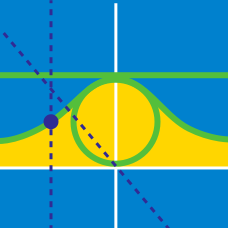Calculus

# Parametric Equations - Velocity and Acceleration

Suppose the position of point $P=(x(t), y(t))$ at time $t$ is given by $\left ( 5{t}^2+4, -5{t}^3+4t \right ) .$ What is the magnitude of acceleration of $P$ at time $t=5 ?$

Suppose the position of point $P=(x, y)$ at time $t$ is given by $\left ( 2t, -2{t}^2+4t \right ).$ What is the magnitude of the velocity of $P$ at time $t=8 ?$

Suppose the position of a particle $P$ at time $t$ is given by $\left ( -8{e}^t\cos t + 2 , 8{e}^t\sin t + 6 \right ).$ What is the angle $\alpha$ ($0 < \alpha < \pi$) between the $x$-axis and the velocity vector $\vec{v}$ of $P$ at time $t= \frac{\pi}{2}?$

Leaving from the origin at the same time, point $P$ moves at a rate of $5$ cm per second in the positive direction of the $x$-axis, while point $Q$ moves at a rate of $10$ cm per second in the positive direction of the $y$-axis. What is the velocity vector $\vec{v}=\left ( \frac{dx}{dt} , \frac{dy}{dt} \right )$ of the intersection point between the line $\overline {PQ}$ and the line $y=3x$?

Suppose the position of point $P=(x(t), y(t))$ at time $t$ is given by $\left ( 4t-10\sin t, 10\cos t + 10 \right ) .$ What is the maximum speed attained by point $P?$

×